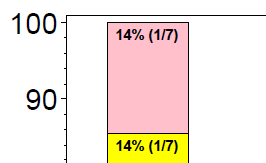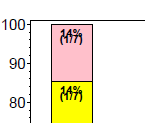## Splitting text in two lines using annotion dataset

Hi,

Is there an option usiung which I can split the text in two lines in an annotation dataset?

Thanks

10 REPLIES 10

## Re: Splitting text in two lines using annotion dataset

You may want to be a bit more explicit about what you are attempting to accomplish.

Do mean is there a way to automatically wrap text? Or to separate the text beforehand and display two variables with the same location information? Or is this to appear in a tool-tip or similar popup?

## Re: Splitting text in two lines using annotion dataset

separate the text beforehand and display two variables with the same location information should solve my problem.

For example,  I am displaying the number 14% (1/7) using the annotation in proc gchart. Is there a way these can be split in two lines?## Re: Splitting text in two lines using annotion dataset

Provide some minimal data in the form of a data step and the code you are currently using and we'll see if we can get what you want. It helps to know what you've done so far.

Modifying the example from http://support.sas.com/kb/45/056.html

The code below 1) adds another statistic 2) modifies the position of the first value and 3) adds value and position for second statistic to the annotate data set

```/* Sum the total sales by product for each country */
proc sort data=sashelp.prdsale out=prdsale;
by product country;
run;
proc means data=prdsale noprint;
by product country;
var actual;
output out=meansout sum=actual_sum std=sTD; /*<= new statistic*/
run;

/* Create an Annotate data set to draw  */
/* the summed values for each subgroup. */
data barlabel;
length function color \$8 text \$ 16;
retain xsys ysys '2' color 'black' when 'a';
set meansout;

function='label';
midpoint=product;
subgroup=country;
position='A'; /*<=change position from original*/
text=trim(left(put(actual_sum,dollar8.0)));
output; /*<= force output of first part of labe text*/
function='label';    /* from here down add second value for same input record*/
midpoint=product;
subgroup=country;
position='D';       /*complementary position to above*/
text=cats('STD=',put(std,dollar8.2));
output;
run;

/* Define the title */
title1 "Label the Subgroups in a Horizontal Bar Chart";

/* Define the axis characteristics */
axis1 label=(angle=90 "Product")
value=(justify=right);
axis2 label=("Actual Sales")
order=(0 to 160000 by 40000)
minor=(n=3);

/* Define legend options */
legend1 frame;

/* Generate the graph */
proc gchart data=sashelp.prdsale;
hbar product / subgroup=country
sumvar=actual
legend=legend1
nostats
maxis=axis1
raxis=axis2
annotate=barlabel;
format actual dollar8.0;
run;
quit; title;```

## Re: Splitting text in two lines using annotion dataset

Thanks your examle code is really helpful. Just one problem, is there a way we can define 1 1/2 cell below center?

I used position='E';  and position='8';  and getting the attached. How can I add space between the lines?

Thanks a lot for your help!!## Re: Splitting text in two lines using annotion dataset

Since E is below and 8 is below I'm not surprised you get a collision. Instead would try 5 and then 2 for the top with 8 in the bottom. If that doesn't quite work I might try a combination of a Left position(1,4,7,A) for the top and then a right position (9 or F)

## Re: Splitting text in two lines using annotion dataset

Thanks but I am little unclear of how to apply combination of positions for example you said

try 5 and then 2 for the top.

- combination of a Left position(1,4,7,A) for the top

## Re: Splitting text in two lines using annotion dataset

Show the code you are using for your annotate data set.

## Re: Splitting text in two lines using annotion dataset

data barlabel;
length function \$8;
retain style '"arial/bo"' xsys ysys '2' when 'a' size 1.5;

set barval;

function='label';
midpoint=dose;
subgroup=avalc;
group=dose;
position='5';
text=strip(round(trim(num)/trim(den)*100) || '%'); output;

function='label';
midpoint=dose;
subgroup=avalc;
group=dose;
position='8';
text='('||strip(num)||'/'||strip(den)||')'; output;
run;

## Re: Splitting text in two lines using annotion dataset

HIJay54
Meteorite | Level 14

## Re: Splitting text in two lines using annotion dataset

If you are using SG annotation with SG procedures, the text string will always get wrapped within the WIDTH parameter on white space.

Discussion stats
• 10 replies
• 2295 views
• 1 like
• 3 in conversation